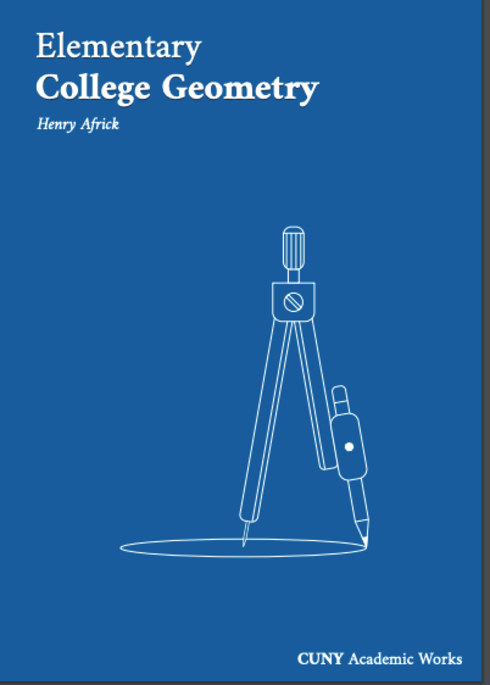# Elementary College Geometry - 2021 ed.

(0 reviews)

No ratings

Henry Africk, New York City College of Technology

Language: English

## Conditions of UseAttribution-NonCommercial-ShareAlike
CC BY-NC-SA

• Preface
• Chapter I Lines Angles And Triangles
• Chapter II Congruent Triangles
• Chapter IV Similar Triangles
• Chapter V Trigonometry Of The Right Triangle
• Chapter VI Area And Perimeter
• Chapter VII Regular Polygons And Circles
• Appendix
• Values Of The Trigonometric Functions
• Answers To Odd Numbered Problems
• Bibliography
• List Of Symbols
• Index

## Ancillary Material

• Submit ancillary resource

This text is intended for a brief introductory course in plane geometry. It covers the topics from elementary geometry that are most likely to be required for more advanced mathematics courses. The only prerequisite is a semester of algebra.

The emphasis is on applying basic geometric principles to the numerical solution of problems. For this purpose the number of theorems and definitions is kept small. Proofs are short and intuitive, mostly in the style of those found in a typical trigonometry or precalculus text. There is little attempt to teach theorem-proving or formal methods of reasoning. However the topics are ordered so that they may be taught deductively.

The problems are arranged in pairs so that just the odd-numbered or just the even-numbered can be assigned. For assistance, the student may refer to a large number of completely worked-out examples. Most problems are presented in diagram form so that the difficulty of translating words into pictures is avoided. Many problems require the solution of algebraic equations in a geometric context. These are included to reinforce the student's algebraic and numerical skills, A few of the exercises involve the application of geometry to simple practical problems. These serve primarily to convince the student that what he or she is studying is useful. Historical notes are added where appropriate to give the student a greater appreciation of the subject.

This book is suitable for a course of about 45 semester hours. A shorter course may be devised by skipping proofs, avoiding the more complicated problems and omitting less crucial topics.